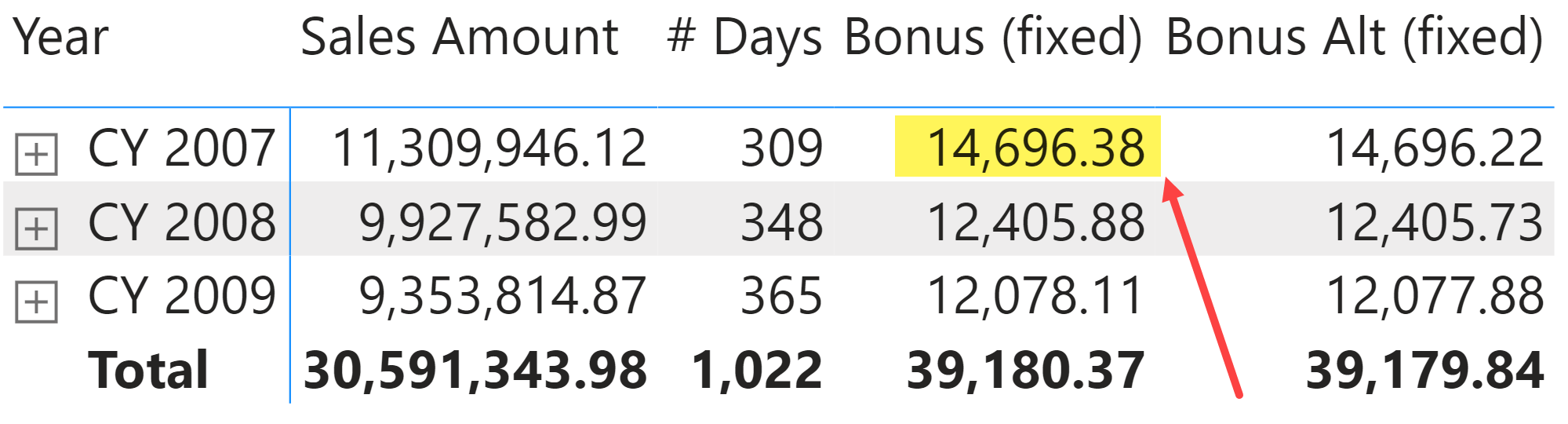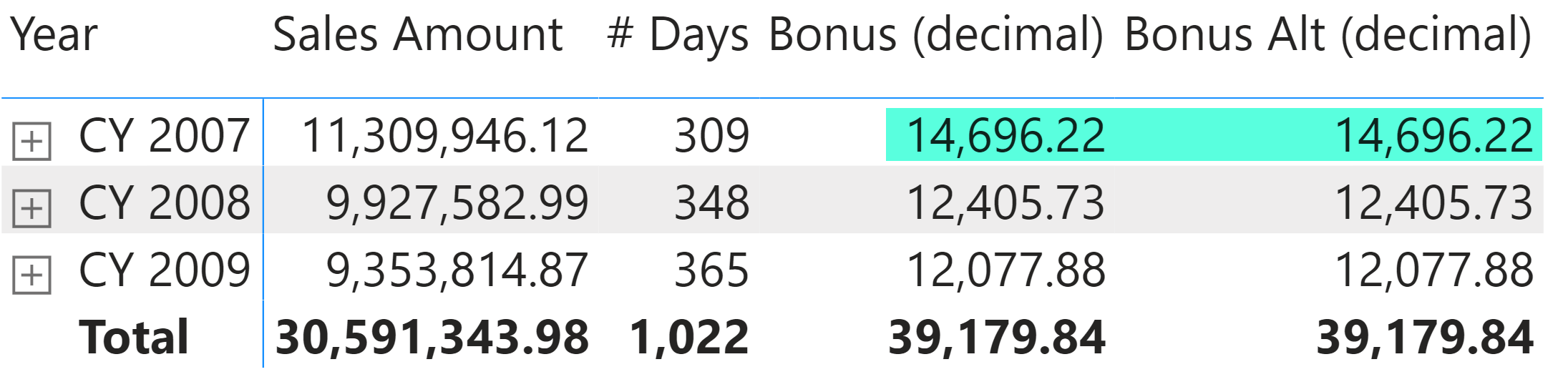It is not common to face rounding differences in Power BI. However, when it happens, users are usually disappointed and surprised.
In one of the last classrooms I delivered, students were wondering why the results of their formulas were close but not identical to the proposed solution. We quickly identified the problem being an issue of data type conversion already covered in Understanding numeric data type conversions in DAX. However, the issue is interesting as a simpler example to show that different DAX calculations can produce different results because of a different way of rounding numbers!

Let’s start with two different implementations of the same business logic: a Bonus measure that computes 0.1% of the sales made on working days and 0.2% of sales made on non-working days. The sales amount for each transaction is obtained by multiplying Sales[Quantity] by Sales[Net Price]. The two measures Bonus (fixed) and Bonus Alt (fixed) implement the same business logic in two different ways:

Measure in Sales table
```Bonus (fixed) :=
SUMX (
Sales,
VAR Amt = Sales[Quantity] * Sales[Net Price]
VAR Pct = IF ( RELATED ( 'Date'[WorkingDay] ) = 1, 0.001, 0.002 )
RETURN
Amt * Pct
)
```
Measure in Sales table
```Bonus Alt (fixed) :=
CALCULATE ( [Sales Amount], 'Date'[WorkingDay] = 1 ) * .001
+
CALCULATE ( [Sales Amount], 'Date'[WorkingDay] = 0 ) * .002
```
Measure in Sales table
```Sales Amount :=
SUMX ( Sales, Sales[Quantity] * Sales[Net Price] )
```

The result is slightly different producing 14,696.38 for Bonus (fixed) and 14,696.22 for Bonus Alt (fixed), with a difference of 0.16 (0.001%) between the two measures.Why is this happening?
The reason is the following: the Sales[Net Price] column has a Fixed Decimal Number data type in Power BI, which corresponds to the Currency data type in DAX (do not confuse data types with format). The result of multiplication between Sales[Quantity] (Whole number data type in Power BI, which is an Integer in DAX) and Sales[Net Price] (Currency) is a Currency data type. Such a result is multiplied by 0.001 or 0.002 depending on the working day state. Because 0.001 and 0.002 are considering Decimal data types in DAX, the result of the multiplication of a Currency multiplied by a Decimal is still a… Currency! It could be counter-intuitive, but it is the way the implicit data type conversion works in multiplications (see Understanding numeric data type conversions in DAX for more details).

The Sales[Net Price] column has numbers with 0.01 granularity. By multiplying 0.01 by 0.001 you get 0.00001, which is outside of the minimum amount that can be represented by a Currency data type in DAX. Therefore, the rounding happens for each transaction in the Sales table, and we have 100,231 transactions in the entire Sales table. Clearly, the rounding could go up and down, depending on the numbers managed. This rounding happens only twice for each cell in the report when we use the Bonus Alt (fixed) measure because the Sales Amount measure computes a sum between Currency data types without any rounding.

What is the right result? While it could be intuitive to say that Bonus Alt (fixed) is better because it limits the number of roundings, there are business scenarios where it is a requirement to perform the rounding for every transaction or for all the transactions of every customer. In those cases, the result produced by Bonus Alt (fixed) would be wrong and should not be used!

Assuming that we want to get the same result produced by Bonus Alt (fixed) also with the algorithm of the Bonus (fixed) measure, we should convert the number into a Decimal before the multiplication that could produce the rounding to a Currency data type. For example, the Bonus (convert) measure produces the same result as Bonus Alt (fixed) because it converts the Amt variable into a Decimal (which is the data type for DOUBLE keyword in the CONVERT function) just before the final multiplication:

Measure in Sales table
```Bonus (convert) =
SUMX (
Sales,
VAR Amt = Sales[Quantity] * Sales[Net Price]
VAR Pct = IF ( RELATED ( 'Date'[WorkingDay] ) = 1, 0.001, 0.002 )
RETURN
CONVERT ( Amt, DOUBLE ) * Pct
)
```

Another approach is that of converting the Sales[Net Price] column into a Decimal Number data type in Power BI. This way the result of the first multiplication Sales[Quantity] * Sales[Net Price] is a Decimal Number, and this keeps the floating-point value in the following multiplications without rounding the number to the 0.0001 precision of the Fixed Decimal Number we had before. The Bonus (decimal) and Bonus Alt (decimal) measures in the sample file implement the same calculations using a Sales[Net Price Decimal] column that has the same value of Sales[Net Price] in a Decimal Number data type. This way we obtain the same value for both measures.The purpose of this blog post is to show a practical example of rounding differences. In my experience, these differences are not relevant and can be ignored. However, when you have to match numbers to the cent, you need to carefully evaluate the data type to use in your calculations. Storing financial values as Fixed Decimal Number is usually a good idea to avoid other rounding issues when you aggregate a large number of rows (like when you see 10.99999999 instead of 11.0). However, when these values are involved in multiplications and divisions, you have to be aware of the possible consequences. In those cases, take a look at the table in the Understanding numeric data type conversions in DAX article to evaluate possible side effects.

CONVERTConvert an expression to the specified data type.

`CONVERT ( <Expression>, <DataType> )`